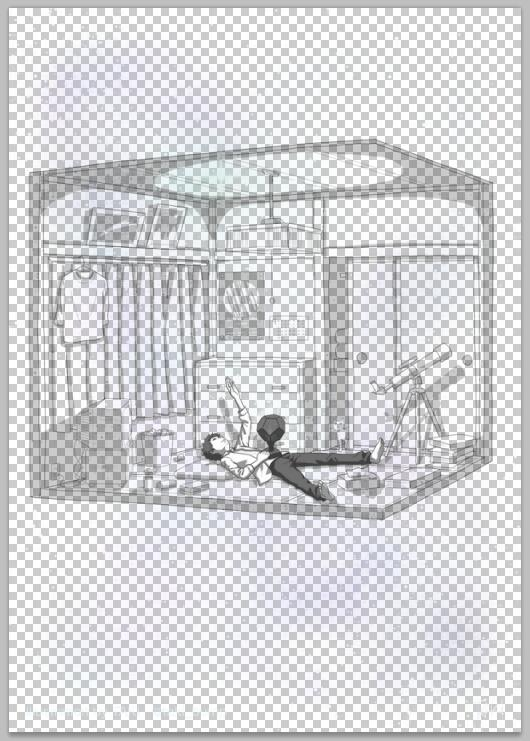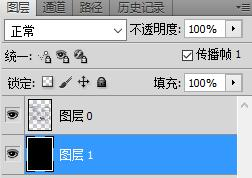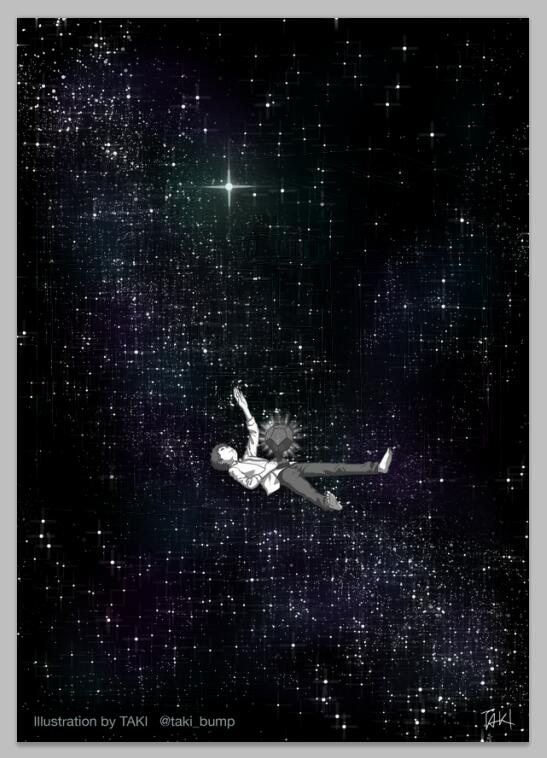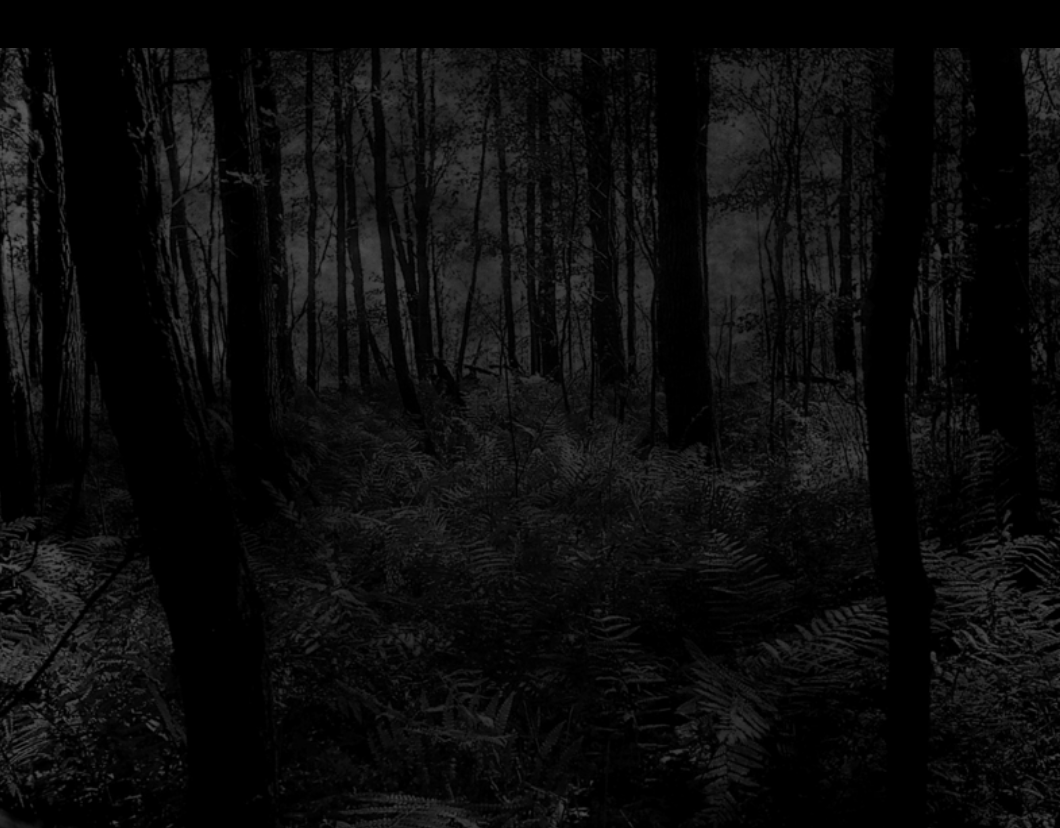# 用Python生成不同背景下显示内容不同的图片$$\begin{equation} \begin{cases} C_1 = C \cdot A + 0 \cdot \left( 1 – A \right) \\ C_2 = C \cdot A + 1 \cdot \left( 1 – A \right) \end{cases} \end{equation}$$

$$\begin{equation} \begin{cases} C = \frac {C_1} {1 + C_1 – C_2} \\ A = 1 + C_1 – C_2 \end{cases} \end{equation}$$

• 两张原始图片分辨率相同
• 想要在黑色背景下显示的图足够暗
• 想要在白色背景下显示的图足够亮
• 输出的图片只有灰度，没有颜色## 《用Python生成不同背景下显示内容不同的图片》有4个想法

1. tecog说道：

没有用到的图片下载差评2333